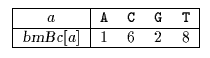Name
• String
• Substring
• Search
Edit

The bad-character shift used in the Boyer-Moore algorithm (see chapter Boyer-Moore algorithm) is not very efficient for small alphabets, but when the alphabet is large compared with the length of the pattern, as it is often the case with the ASCII table and ordinary searches made under a text editor, it becomes very useful.
Using it alone produces a very efficient algorithm in practice. Horspool proposed to use only the bad-character shift of the rightmost character of the window to compute the shifts in the Boyer-Moore algorithm.

The preprocessing phase is in O(m+) time and O() space complexity.

The searching phase has a quadratic worst case but it can be proved that the average number of comparisons for one text character is between 1/and 2/(+1).

Main features:

• simplification of the Boyer-Moore algorithm;
• easy to implement;
• preprocessing phase in O(m+) time and O() space complexity;
• searching phase in O(mn) time complexity;
• the average number of comparisons for one text character is between 1/ and 2/(+1).

Example:

Preprocessing phasebmBc table used by Horspool algorithm

Searching phase

 G C A T C G C A G A G A G T A T A C A G T A C G 1 G C A G A G A G

Shift by: 1 (bmBc[A])

 G C A T C G C A G A G A G T A T A C A G T A C G 2 1 G C A G A G A G

Shift by: 2 (bmBc[G])

 G C A T C G C A G A G A G T A T A C A G T A C G 2 1 G C A G A G A G

Shift by: 2 (bmBc[G])

 G C A T C G C A G A G A G T A T A C A G T A C G 2 3 4 5 6 7 8 1 G C A G A G A G

Shift by: 2 (bmBc[G])

 G C A T C G C A G A G A G T A T A C A G T A C G 1 G C A G A G A G

Shift by: 1 (bmBc[A])

 G C A T C G C A G A G A G T A T A C A G T A C G 1 G C A G A G A G

Shift by: 8 (bmBc[T])

 G C A T C G C A G A G A G T A T A C A G T A C G 2 1 G C A G A G A G

Shift by: 2 (bmBc[G])

The Horspool algorithm performs 17 character comparisons on the example.

C